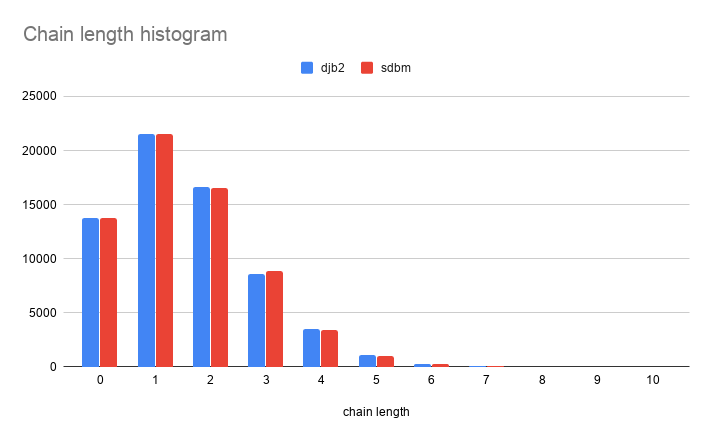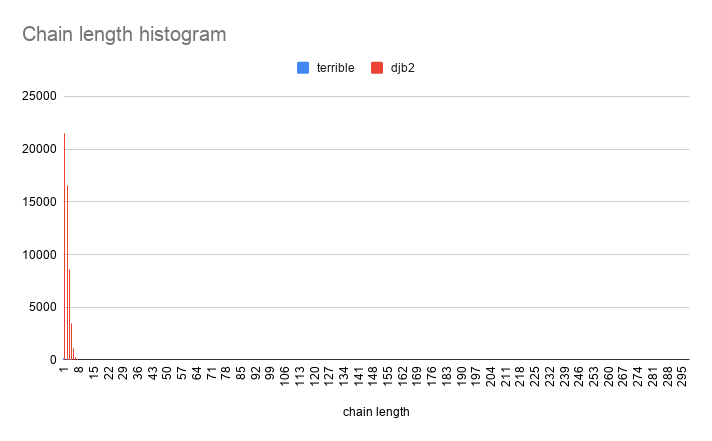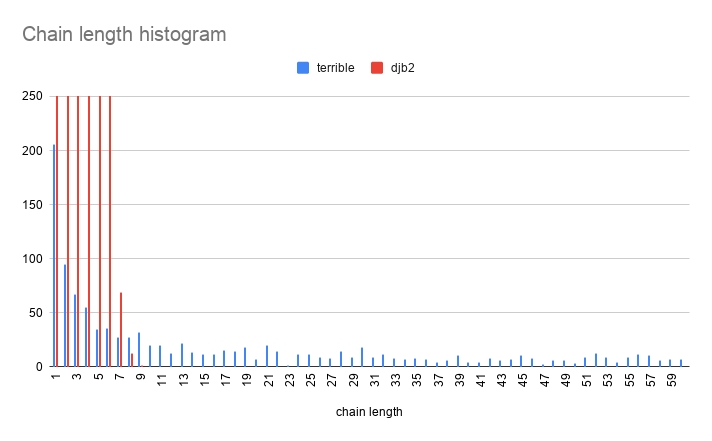Instantly share code, notes, and snippets.

# cellularmitosis/HashTable.c

Last active July 29, 2021 18:53
Hash table in C, part 5: better hashing

Blog 2020/8/21

# Hash table in C, part 5: better hashing

Let's learn how to implement a hash table in C! (See also part 1, 2, 3 and 4).

Let's take a look at the importance of the hash function by comparing "terrible hash" to DJB2 and SDBM

```size_t djb2Hash(void* datap, size_t length) {
size_t hash = 5381;
uint8_t* cursorp = datap;
for (size_t i=0; i < length; cursorp++, i++) {
hash = ((hash << 5) + hash) ^ (*cursorp);
}
return hash;
}```
```size_t sdbmHash(void* datap, size_t length) {
size_t hash = 0;
uint8_t* cursorp = datap;
for (size_t i=0; i < length; cursorp++, i++) {
hash = (*cursorp) + (hash << 6) + (hash << 16) - hash;
}
return hash;
}```

## Hashing the dictionary

To analyze the performance of these hash functions, we'll add all of the words (about 100,000) in `/usr/share/dict/words` as keys in a hash table, then look at a histogram of slot list lengths.

We'll use a function pointer so that we can easily pass in different hashing functions.

```HashTable* hashTheDictionary(size_t (*hashFuncp)(void*, size_t)) {
// open the dictionary file
int fd = open("/usr/share/dict/words", O_RDONLY);
assert(fd != -1);

// get the size of the file
struct stat statbuf;
int err = fstat(fd, &statbuf);
assert(err == 0);
off_t fsize = statbuf.st_size;

// read the file into memory.
char* fbuff = (char*)malloc(fsize);
assert(fbuff != NULL);

HashTable* htp = HashTableMake(1);
char* valuep = "common value";
char* cursorp = fbuff;
char* endp = fbuff + fsize;
char* keyp = cursorp;
while (cursorp != endp) {
if (*cursorp == '\n') {
*cursorp = '\0';
size_t keyLength = cursorp - keyp;
if (keyLength > 0) {
size_t keyHash = hashFuncp(keyp, keyLength);
HashTableSet(htp, keyp, keyLength, keyHash, valuep);
}
cursorp++;
keyp = cursorp;
continue;
} else {
cursorp++;
continue;
}
}

return htp;
}```
```void analyzeHashTable(HashTable* htp) {
#define LENGTHS_LEN (1000)
size_t score = 0;
size_t lengths[LENGTHS_LEN] = {0};
for (size_t i=0; i < htp->capacity; i++) {
HTSlot* slotp = htp->slotspp[i];
size_t chainLength = 0;
while (slotp != NULL) {
if (slotp->valuep != NULL) {
chainLength++;
}
slotp = slotp->nextp;
continue;
}
lengths[chainLength]++;
score += (chainLength * chainLength);
continue;
}

printf("Chain lengths histogram:\n");
printf("length occurrences\n");
printf("------ -----------\n");
for (size_t i=0; i < LENGTHS_LEN; i++) {
if (lengths[i] == 0) {
continue;
}

if (i < 10) {
printf("     ");
} else if (i < 100) {
printf("    ");
} else if (i < 1000) {
printf("   ");
} else if (i < 10000) {
printf("  ");
} else if (i < 100000) {
printf(" ");
}
printf("%zu %zu\n", i, lengths[i]);
}
printf("population: %zu, slots: %zu, empty slots: %zu, score: %zu\n",
htp->population, htp->capacity, lengths, score
);
}```
```int main(int argc, char** argv) {
HashTable* htp;

printf("\ndjb2 hash\n");
htp = hashTheDictionary(djb2Hash);
analyzeHashTable(htp);

printf("\nsdbm hash\n");
htp = hashTheDictionary(sdbmHash);
analyzeHashTable(htp);

printf("\nterrible hash\n");
htp = hashTheDictionary(terribleHash);
analyzeHashTable(htp);

return 0;
}```

## Results

What we are looking for is a "short tail". The ideal hash function would give us an even distribution of hash values. That is, if we had N slots and N keys, all of our slot lists would be length 1.

Practically, some slots will be empty and others will be length > 1.

### terrible hash

First, let's take a look at "terrible hash":

``````terrible hash
Chain lengths histogram:
length occurrences
------ -----------
0 63741
1 206
2 95
3 67
4 55
5 35
6 36
7 28
8 28
9 32
10 20
11 20
12 13
13 22
14 14
15 12
(...many rows elided...)
82 8
83 10
84 12
85 7
86 11
87 6
88 8
89 2
90 6
91 5
(...many rows elided...)
266 1
267 1
268 2
269 2
272 2
274 1
279 1
283 1
288 2
298 1
population: 102401, slots: 65536, empty slots: 63741, score: 13240151
``````

Oof! This is truly terrible! 97% of the slots are unused, and one of the slots has a chain which is 298 elements long!!!

This hash produces a long tail, which is the opposite of what we want!

### DJB2 and SDBM

Now let's compare that to DJB2 and SDBM.

``````DJB2 hash
Chain lengths histogram:
length occurrences
------ -----------
0 13809
1 21547
2 16591
3 8633
4 3484
5 1096
6 292
7 69
8 13
9 2
population: 102401, slots: 65536, empty slots: 13809, score: 263639
``````
``````SDBM hash
Chain lengths histogram:
length occurrences
------ -----------
0 13778
1 21522
2 16539
3 8841
4 3447
5 1056
6 275
7 66
8 11
10 1
population: 102401, slots: 65536, empty slots: 13778, score: 262737
``````

Visually:DBJ2 and SDBM are remarkably similar in distribution!

Now let's compare DJB2 to "terrible hash" :)The difference in performance is so severe that they can't really be compared on the same graph! Let's zoom in:## Next time

In part 6, we'll take a look at using prime numbers for our slot array sizes.

This file contains bidirectional Unicode text that may be interpreted or compiled differently than what appears below. To review, open the file in an editor that reveals hidden Unicode characters. Learn more about bidirectional Unicode characters
 #include "HashTable.h" #include // fprintf #include // exit #include // memset #include // uint8_t #include // true #include // assert void* dmalloc(size_t size) { void* p = malloc(size); if (p == NULL) { perror("Error: malloc failed: "); exit(1); } memset(p, 0, size); return p; } #define malloc(x) dont_use_malloc_use_dmalloc size_t terribleHash(void* datap, size_t length) { size_t sum = 0; for (size_t i=0; i < length; i++) { uint8_t* byte = datap + i; sum += *byte; continue; } return sum; } size_t djb2Hash(void* datap, size_t length) { size_t hash = 5381; uint8_t* cursorp = datap; for (size_t i=0; i < length; cursorp++, i++) { hash = ((hash << 5) + hash) ^ (*cursorp); } return hash; } size_t sdbmHash(void* datap, size_t length) { size_t hash = 0; uint8_t* cursorp = datap; for (size_t i=0; i < length; cursorp++, i++) { hash = (*cursorp) + (hash << 6) + (hash << 16) - hash; } return hash; } static HTSlot* _HTSlotMake() { HTSlot* sp = dmalloc(sizeof(HTSlot)); return sp; } HashTable* HashTableMake(size_t capacity) { HashTable* htp = dmalloc(sizeof(HashTable)); htp->capacity = capacity; htp->minCapacity = capacity; htp->slotspp = dmalloc(sizeof(void*) * capacity); return htp; } void HashTableFree(HashTable* htp) { for (size_t i=0; i < htp->capacity; i++) { HTSlot* slotp = htp->slotspp[i]; while (slotp != NULL) { HTSlot* tmp = slotp; free(slotp); slotp = tmp->nextp; continue; } } free(htp->slotspp); free(htp); } void* HashTableGet(HashTable* htp, void* keyp, size_t keyLength, size_t keyHash ) { size_t slotIndex = keyHash % htp->capacity; HTSlot* cursorp = htp->slotspp[slotIndex]; while (cursorp != NULL) { if (cursorp->keyHash == keyHash && cursorp->keyLength == keyLength && memcmp(cursorp->keyp, keyp, keyLength) == 0 ) { return cursorp->valuep; } else { cursorp = cursorp->nextp; continue; } } return NULL; } static void _adjustCapacityIfNeeded(HashTable* htp); void HashTableSet(HashTable* htp, void* keyp, size_t keyLength, size_t keyHash, void* valuep ) { size_t origPop = htp->population; size_t slotIndex = keyHash % htp->capacity; HTSlot* cursorp = htp->slotspp[slotIndex]; while (cursorp != NULL) { if (cursorp->keyHash == keyHash && cursorp->keyLength == keyLength && memcmp(cursorp->keyp, keyp, keyLength) == 0 ) { if (cursorp->valuep == NULL && valuep != NULL) { htp->population++; } else if (cursorp->valuep != NULL && valuep == NULL) { htp->population--; } cursorp->valuep = valuep; goto adjust; } else { cursorp = cursorp->nextp; continue; } } // no existing match? append a new slot to the list. if (valuep != NULL) { HTSlot* newSlotp = _HTSlotMake(); newSlotp->keyp = keyp; newSlotp->keyLength = keyLength; newSlotp->keyHash = keyHash; newSlotp->valuep = valuep; if (htp->slotspp[slotIndex] == NULL) { htp->slotspp[slotIndex] = newSlotp; } else { HTSlot* lastp = htp->slotspp[slotIndex]; while (lastp->nextp != NULL) { lastp = lastp->nextp; continue; } lastp->nextp = newSlotp; } htp->population++; } adjust: if (origPop != htp->population) { _adjustCapacityIfNeeded(htp); } } static bool _shouldShrink(HashTable* htp) { return htp->capacity >= (htp->population * 2); } static bool _shouldGrow(HashTable* htp) { return htp->population >= (htp->capacity * 2); } static void _adjustCapacityIfNeeded(HashTable* htp) { size_t newCapacity; if (_shouldShrink(htp)) { newCapacity = htp->capacity / 2; } else if (_shouldGrow(htp)) { newCapacity = htp->capacity * 2; } else { return; } if (newCapacity < htp->minCapacity) { return; } HTSlot** slots2pp = dmalloc(sizeof(void*) * newCapacity); for (size_t i=0; i < htp->capacity; i++) { HTSlot* cursorp = htp->slotspp[i]; while (cursorp != NULL) { HTSlot* tmp = cursorp; cursorp = cursorp->nextp; if (tmp->valuep != NULL) { tmp->nextp = NULL; size_t slotIndex = tmp->keyHash % newCapacity; if (slots2pp[slotIndex] == NULL) { slots2pp[slotIndex] = tmp; } else { HTSlot* lastp = slots2pp[slotIndex]; while (lastp->nextp != NULL) { lastp = lastp->nextp; continue; } lastp->nextp = tmp; } } continue; } } free(htp->slotspp); htp->slotspp = slots2pp; htp->capacity = newCapacity; }
This file contains bidirectional Unicode text that may be interpreted or compiled differently than what appears below. To review, open the file in an editor that reveals hidden Unicode characters. Learn more about bidirectional Unicode characters
 #ifndef _HASHTABLE_H_ #define _HASHTABLE_H_ #include // size_t size_t terribleHash(void* datap, size_t length); size_t djb2Hash(void* datap, size_t length); size_t sdbmHash(void* datap, size_t length); struct _HTSlot { void* keyp; size_t keyLength; size_t keyHash; void* valuep; struct _HTSlot* nextp; }; typedef struct _HTSlot HTSlot; struct _HashTable { HTSlot** slotspp; size_t capacity; size_t population; size_t minCapacity; }; typedef struct _HashTable HashTable; HashTable* HashTableMake(size_t capacity); void HashTableFree(HashTable* htp); void* HashTableGet(HashTable* htp, void* keyp, size_t keyLength, size_t keyHash ); void HashTableSet(HashTable* htp, void* keyp, size_t keyLength, size_t keyHash, void* valuep ); #endif
This file contains bidirectional Unicode text that may be interpreted or compiled differently than what appears below. To review, open the file in an editor that reveals hidden Unicode characters. Learn more about bidirectional Unicode characters
 #include // open #include // assert #include // malloc #include // fstat #include #include // read #include // printf #include // uint8_t #include "HashTable.h" HashTable* hashTheDictionary(size_t (*hashFuncp)(void*, size_t)) { // open the dictionary file int fd = open("/usr/share/dict/words", O_RDONLY); assert(fd != -1); // get the size of the file struct stat statbuf; int err = fstat(fd, &statbuf); assert(err == 0); off_t fsize = statbuf.st_size; // read the file into memory. char* fbuff = (char*)malloc(fsize); assert(fbuff != NULL); ssize_t bytesRead = read(fd, fbuff, fsize); assert(bytesRead == fsize); HashTable* htp = HashTableMake(1); char* valuep = "common value"; char* cursorp = fbuff; char* endp = fbuff + fsize; char* keyp = cursorp; while (cursorp != endp) { if (*cursorp == '\n') { *cursorp = '\0'; size_t keyLength = cursorp - keyp; if (keyLength > 0) { size_t keyHash = hashFuncp(keyp, keyLength); HashTableSet(htp, keyp, keyLength, keyHash, valuep); } cursorp++; keyp = cursorp; continue; } else { cursorp++; continue; } } return htp; } void analyzeHashTable(HashTable* htp) { #define LENGTHS_LEN (1000) size_t score = 0; size_t lengths[LENGTHS_LEN] = {0}; for (size_t i=0; i < htp->capacity; i++) { HTSlot* slotp = htp->slotspp[i]; size_t chainLength = 0; while (slotp != NULL) { if (slotp->valuep != NULL) { chainLength++; } slotp = slotp->nextp; continue; } lengths[chainLength]++; score += (chainLength * chainLength); continue; } printf("Chain lengths histogram:\n"); printf("length occurrences\n"); printf("------ -----------\n"); for (size_t i=0; i < LENGTHS_LEN; i++) { if (lengths[i] == 0) { continue; } if (i < 10) { printf(" "); } else if (i < 100) { printf(" "); } else if (i < 1000) { printf(" "); } else if (i < 10000) { printf(" "); } else if (i < 100000) { printf(" "); } printf("%zu %zu\n", i, lengths[i]); } printf("population: %zu, slots: %zu, empty slots: %zu, score: %zu\n", htp->population, htp->capacity, lengths, score ); } int main(int argc, char** argv) { HashTable* htp; printf("\ndjb2 hash\n"); htp = hashTheDictionary(djb2Hash); analyzeHashTable(htp); printf("\nsdbm hash\n"); htp = hashTheDictionary(sdbmHash); analyzeHashTable(htp); printf("\nterrible hash\n"); htp = hashTheDictionary(terribleHash); analyzeHashTable(htp); return 0; }
This file contains bidirectional Unicode text that may be interpreted or compiled differently than what appears below. To review, open the file in an editor that reveals hidden Unicode characters. Learn more about bidirectional Unicode characters
 default: tests ./tests tests: HashTable.o tests.c gcc -g -std=c99 -Wall -Werror HashTable.o tests.c -o tests histograms: HashTable.o histograms.c gcc -g -std=c99 -Wall -Werror HashTable.o histograms.c -o histograms HashTable.o: HashTable.h HashTable.c gcc -g -std=c99 -Wall -Werror -c HashTable.c clean: rm -f HashTable.o tests histograms .PHONY: default clean
This file contains bidirectional Unicode text that may be interpreted or compiled differently than what appears below. To review, open the file in an editor that reveals hidden Unicode characters. Learn more about bidirectional Unicode characters
 #include "HashTable.h" #include // assert #include // printf #include // strlen, strcmp void testMake() { printf("%s... ", __FUNCTION__); size_t capacity = 3; HashTable* htp = HashTableMake(capacity); assert(htp != NULL); assert(htp->capacity == 3); printf("OK\n"); } void testFree() { printf("%s... ", __FUNCTION__); size_t capacity = 3; HashTable* htp = HashTableMake(capacity); HashTableFree(htp); printf("OK\n"); } void testSet() { printf("%s... ", __FUNCTION__); size_t capacity = 3; HashTable* htp = HashTableMake(capacity); char* keyp = "hello"; size_t keyLength = strlen(keyp) + 1; size_t keyHash = terribleHash(keyp, keyLength); char* valuep = "world"; HashTableSet(htp, keyp, keyLength, keyHash, valuep); assert(htp->population == 1); printf("OK\n"); } void testGet() { printf("%s... ", __FUNCTION__); size_t capacity = 3; HashTable* htp = HashTableMake(capacity); char* keyp = "hello"; size_t keyLength = strlen(keyp) + 1; size_t keyHash = terribleHash(keyp, keyLength); char* valuep = "world"; HashTableSet(htp, keyp, keyLength, keyHash, valuep); char* gotp = HashTableGet(htp, keyp, keyLength, keyHash); assert(strcmp(valuep, gotp) == 0); printf("OK\n"); } void testSlotCollision() { printf("%s... ", __FUNCTION__); size_t capacity = 1; HashTable* htp = HashTableMake(capacity); char* key1p = "hello"; size_t key1Length = strlen(key1p) + 1; size_t key1Hash = terribleHash(key1p, key1Length); char* value1p = "world"; HashTableSet(htp, key1p, key1Length, key1Hash, value1p); assert(htp->population == 1); char* key2p = "foo"; size_t key2Length = strlen(key2p) + 1; size_t key2Hash = terribleHash(key2p, key2Length); char* value2p = "bar"; HashTableSet(htp, key2p, key2Length, key2Hash, value2p); assert(htp->population == 2); char* got1p = HashTableGet(htp, key1p, key1Length, key1Hash); assert(strcmp(value1p, got1p) == 0); char* got2p = HashTableGet(htp, key2p, key2Length, key2Hash); assert(strcmp(value2p, got2p) == 0); printf("OK\n"); } void testHashCollision() { printf("%s... ", __FUNCTION__); size_t capacity = 1; HashTable* htp = HashTableMake(capacity); char* key1p = "ab"; size_t key1Length = strlen(key1p) + 1; size_t key1Hash = terribleHash(key1p, key1Length); char* value1p = "cd"; HashTableSet(htp, key1p, key1Length, key1Hash, value1p); assert(htp->population == 1); char* key2p = "ba"; size_t key2Length = strlen(key2p) + 1; size_t key2Hash = terribleHash(key2p, key2Length); char* value2p = "dc"; HashTableSet(htp, key2p, key2Length, key2Hash, value2p); assert(htp->population == 2); char* got1p = HashTableGet(htp, key1p, key1Length, key1Hash); assert(strcmp(value1p, got1p) == 0); char* got2p = HashTableGet(htp, key2p, key2Length, key2Hash); assert(strcmp(value2p, got2p) == 0); printf("OK\n"); } void testGrow() { printf("%s... ", __FUNCTION__); size_t capacity = 1; HashTable* htp = HashTableMake(capacity); assert(htp->population == 0); assert(htp->capacity == capacity); char* key1p = "hello"; size_t key1Length = strlen(key1p) + 1; size_t key1Hash = terribleHash(key1p, key1Length); char* value1p = "world"; HashTableSet(htp, key1p, key1Length, key1Hash, value1p); assert(htp->population == 1); assert(htp->capacity == capacity); char* key2p = "foo"; size_t key2Length = strlen(key2p) + 1; size_t key2Hash = terribleHash(key2p, key2Length); char* value2p = "bar"; HashTableSet(htp, key2p, key2Length, key2Hash, value2p); assert(htp->population == 2); assert(htp->capacity == capacity*2); printf("OK\n"); } void testShrink() { printf("%s... ", __FUNCTION__); size_t capacity = 1; HashTable* htp = HashTableMake(capacity); assert(htp->population == 0); assert(htp->capacity == capacity); char* key1p = "hello"; size_t key1Length = strlen(key1p) + 1; size_t key1Hash = terribleHash(key1p, key1Length); char* value1p = "world"; HashTableSet(htp, key1p, key1Length, key1Hash, value1p); assert(htp->population == 1); assert(htp->capacity == capacity); char* key2p = "foo"; size_t key2Length = strlen(key2p) + 1; size_t key2Hash = terribleHash(key2p, key2Length); char* value2p = "bar"; HashTableSet(htp, key2p, key2Length, key2Hash, value2p); assert(htp->population == 2); assert(htp->capacity == capacity*2); HashTableSet(htp, key2p, key2Length, key2Hash, NULL); assert(htp->population == 1); assert(htp->capacity == capacity); printf("OK\n"); } int main(int argc, char** argv) { testMake(); testFree(); testSet(); testGet(); testSlotCollision(); testHashCollision(); testGrow(); testShrink(); return 0; }